Pull Down & Release to Refresh

# Ratios

Ratios compare the values of things. They tell us how much of one thing is made up of another thing, or how much of one thing there is compared to another thing.

For example,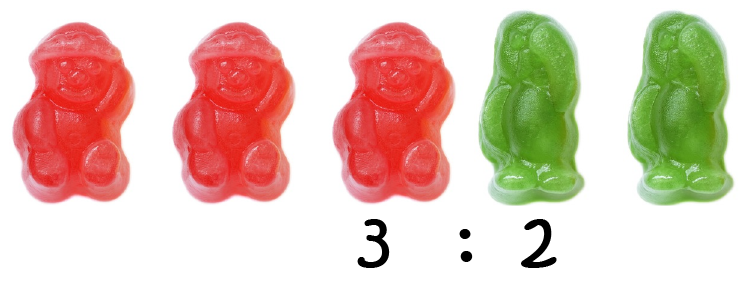in the picture, there are 3 red jelly babies and 2 green jelly babies. The ratio of red to green jelly babies is $3:2$, but we can soon fix that (I love red jelly babies)!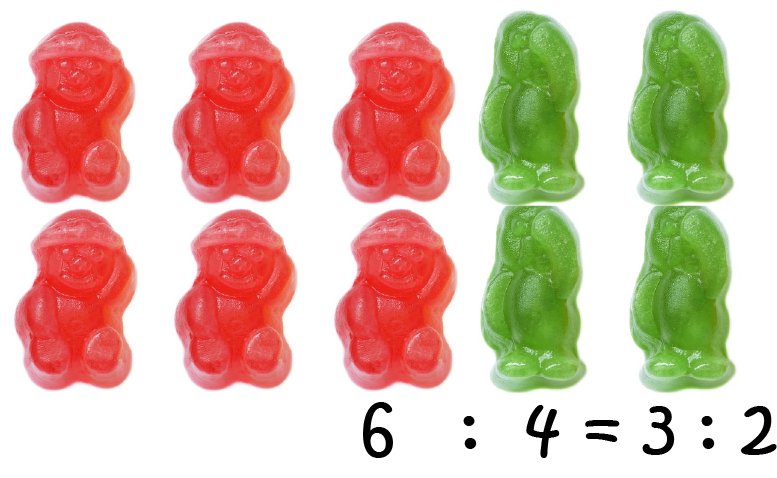There are 6 red jelly babies to 4 red jelly babies, so the ratio of red to green jelly babies is $6:4$. But this simplifies to $3:2$ as both $6$ and $4$ have a factor of $2$, so we can divide both sides of the ratio by $2$.

## Simplifying (or complicating) Ratios

We can make equivalent ratios in much the same way as we make equivalent fractions. We just have to make sure we multiply (or divide) both parts of the ratio by the same amount.

For example, $6:9$ is the same as $6 \times 3: 9 \times 3 = 18:27$ and the same as $6 \div 3 : 9 \div 3 = 2: 3$.

## Example: A Recipe for Puffed Rice Crackles

Christo and Sam have a lot of allergies, so we need to make our own, allergy-free, versions of many foods. Here's a recipe they particularly like for a treat.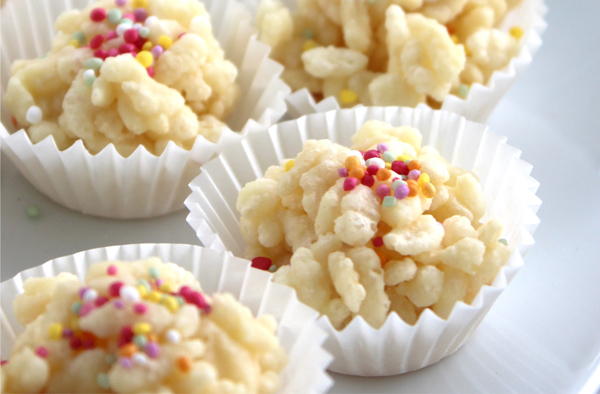Ingredients

• 200 g (white) marshmallows
• 80 g butter
• 120 g puffed rice (use the crunchy stuff, not the big puffy pillowy stuff)

Method

1. Place the puffed rice in a large bowl.
2. Melt marshmallows and butter over low heat, stirring constantly.
3. Pour marshmallow and butter mixture into bowl with puffed rice and stir thoroughly.
4. Spoon into patty pans, and store in the fridge.

I'd forgotten how simple that was. I think I'll make some this weekend.

So, why have I put this recipe in the middle of an article on ratios? I'm glad you asked. The reason is that we can use it to find some ratios.

### Example

What is the ratio of butter to marshmallows in the Puffed Rice Crackles Recipe?

Solution:

There are $80$ g of butter and $200$ g of marshmallows, so the ratio is

$80: 200 = 2 \times 40 : 5 \times 40 = 2:5$.

### Example

What is the ratio of marshmallows to puffed rice in the Puffed Rice Crackles Recipe?

Solution:

There are $200$ g of marshmallows and $120$ g of puffed rice, so the ratio is

$200: 120 = 5 \times 40 : 3 \times 40 = 5:3$.

We can combine these two ratios to give a ratio of the three ingredients butter : marshmallows : puffed rice of $2:5:3$.

This ratio can be used to work out the quantities of butter, marshmallows and puffed rice if you only have a limited amount of one of these ingredients.

For example, if I only have $100$ g of marshmallows, I know that I need $100 \div 5 = 20$ g for each part of each ingredient in the recipe, so I need $2 \times 20 = 40) g of butter and \(3 \times 20 = 60$ g of puffed rice to make some puffed rice crackles.

Of course, if I want to make a triple quantity of the recipe, I can multiply the original quantities by $3$ to give requirements of $600$ g of marshmallows, $360$ g of puffed rice and $240$ g of butter, and I can also multiply each part of the ratio $80:200:120$ by $3$. I still keep my ingredients in the same ratio, so my puffed rice crispies will taste the same.

### Ratio and Proportion

In our previous examples, we've only found ratios of part of something to another part of something.

A proportion is a ratio of a part to a whole. In our rice bubble crackle recipe, the proportion of marshmallows is $200: 200 + 120 + 80 = 200: 400 = 1:2$.

In the jelly baby examples, the proportion of red jelly babies is $3:3 +2 = 3:5$. Yes, I like red jelly babies the best.

### One for You to Do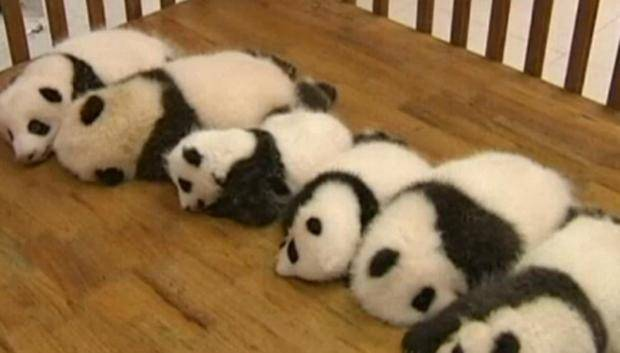A wildlife sanctuary has 14 panda cubs. 6 of them are boys and 8 are girls.

1. What proportion of the panda cubs are girls?
2. What is the ratio of girl cubs to boy cubs?

### Scale Models and Scale Drawings

We can use ratios to make scale drawings or models of real-life objects. This has the advantage that we can fit them on paper, or in our display cabinet, but we still maintain the proportions of the original objects.

#### Example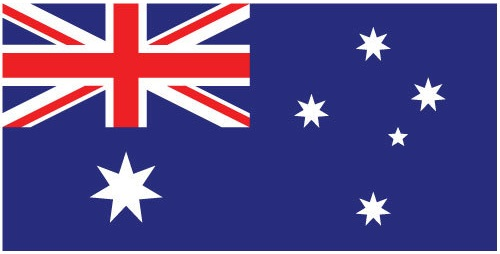The Australian flag must officially have a height to width ratio of $1:2$. This means that, for every $1$ cm of height of the flag, there must be $2$ cm of width.

An Australian flag that is $50$ cm high must be $100$ cm wide.

An Australian flag that is $60$ cm wide must be $30$ cm high.

Let's look at one last example. This one is about the models that Sam and Christo like to build.

#### Example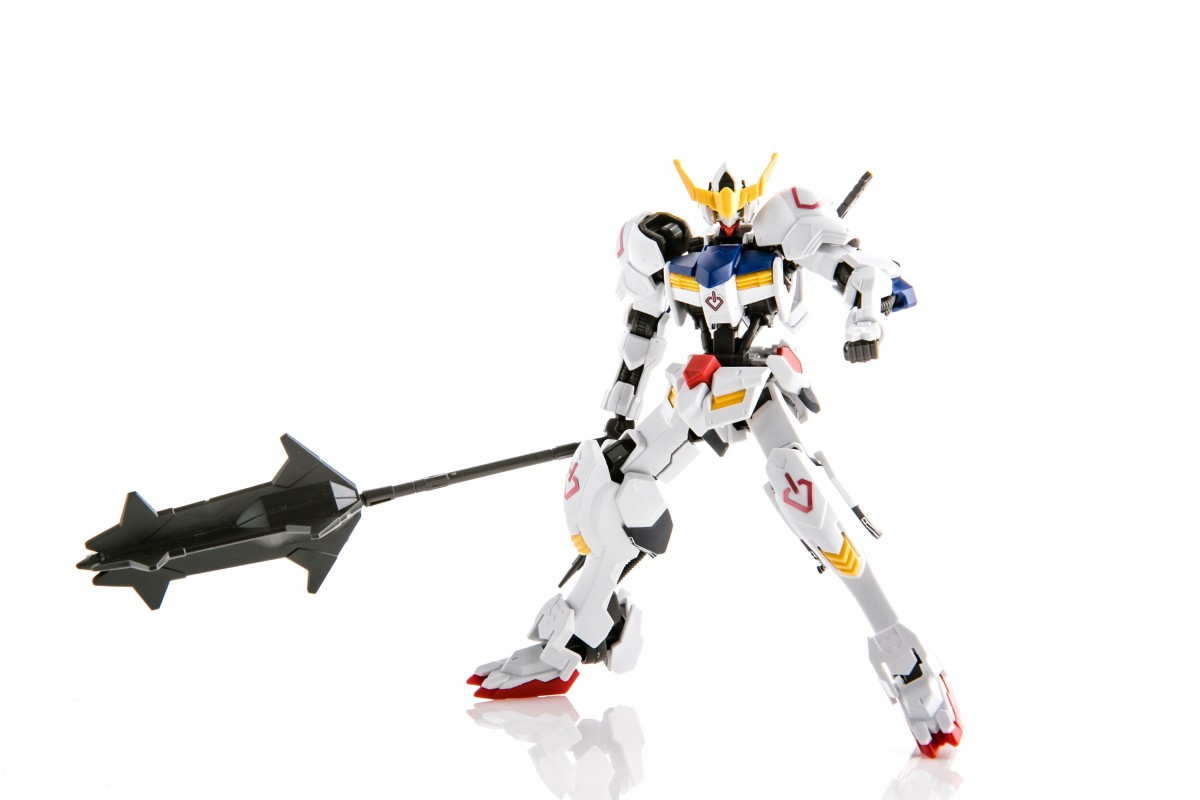Gundam are mechanoid suits from a Japanese anime series. Sam and Christo love building models of these suits. Although they are only really found in the anime series, the suits are supposed to be $18.3$ m high in real life. Obviously, models that big wouldn't fit into our display cabinet, but scale models will.

How tall is a model gundam built at a scale of $1:100$?

This means that every 1 m of height of the "real" gundam corresponds to $\dfrac{1}{100} \times 1 = 0.01 \text{ m}$ of model height. So, the model is $\dfrac{1}{100} \times 18.3 = 0.183 \text{ m}$ high, which is $18.3 \text{ cm}$ high. That's a bit more realistic for showing in a display cabinet!

### Description

In this mini series, you will learn a bit more on the topic of probability, we will cover topics such as

• Ratios
• Fair dice
• Conditional probability
• Mutually exclusive events

and more

### Audience

Year 10 or higher students

### Learning Objectives

Explore more on the topic of probability

Author: Subject Coach
You must be logged in as Student to ask a Question.